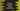# Python program to convert key-value string to dictionary## Python key-value string to dictionary:

Suppose, a string with key-value pairs is given. We need to convert it to a dictionary.

For example, if the string is ‘one: 1, two: 2, three: 3’, and we need to convert it to a dictionary {‘one’: ‘1’, ‘two’: ‘2’, ‘three’: ‘3’}. We can also have strings with any other separated characters.

This post will show you how to do this in Python with example.

### By using split:

We can split the string at , and other items at :. We can then use dictionary comprehension dict() to convert each pair to a dictionary. For example:

``````given_str = 'one: 1, two: 2, three: 3'

splitted_str = given_str.split(',')

dict = {}

for item in splitted_str:
key_value = item.split(':')
dict[key_value] = key_value.strip()

print(dict)``````

Here,

• We are splitting the string two times. The first time, it splits at , and puts the array in splittedstr_.
• It iterates through each item of the splitted array and splits each at :.
• Then it assigns the key-value pairs in the dictionary.

If you run the above program, it will print:

``{'one': '1', ' two': '2', ' three': '3'}``

We can also write this as like below:

``````given_str = 'one: 1, two: 2, three: 3'

final_dict = dict(item.split(":") for item in given_str.split(","))

final_dict = {key.strip(): value.strip()
for (key, value) in final_dict.items()}

print(final_dict)``````

The first line is enough to create the finaldict, but we are also removing the _leading and trailing spaces by using strip() in the second line.

It will print the same output.

### Using map:

We can also pass a lambda to a map to create the key-value pairs. It can be passed to dictionary comprehension to get the final dictionary. Below is the complete program:

``````given_str = 'one: 1, two: 2, three: 3'

final_dict = dict(map(lambda item: item.split(':'), given_str.split(',')))

final_dict = {key.strip(): value.strip()
for (key, value) in final_dict.items()}

print(final_dict)``````

It gives the same output.